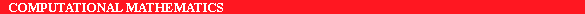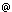ERCIM News No.22 - July 1995 - CWI

# Multigrid, Semi-refinement and Fluid Flow

by Piet Hemker

Multigrid methods have shown their ability to produce fast and efficient solutions of partial differential equations. CWI's considerable experience with these methods is presently applied to three-dimensional fluid flow problems.

Partial differential equations (PDEs) are numerically solved by discretization and solving the resulting system of algebraic equations on a computer. In practical situations, for example the accurate solution of a 3D problem, the size of this algebraic system can be enormous. Among the special techniques, which have been developed to provide practical solutions of such large systems, the multigrid (MG) method has acquired a prominent place. Invented in the 1960s by the Russians Fedorenko and Bakhvalov, it got full attention only in the 1980s and is presently well-accepted and successfully applied in many fields. The method is optimal in the sense that it is the only approach by which the amount of computational work to solve the algebraic system grows only proportional to N, the number of unknowns. All other known methods have a faster growth. For example, in solving the Poisson equation on a square by Gauss elimination, the number of arithmetic operations is proportional to N 3.

The usual procedure to solve PDEs numerically is by iteration. Relaxation methods are a special, simple form of iteration. The equations are solved on one grid point for the variables associated with that point, keeping all other variables fixed. This is done for all grid points (one `sweep'). As a result the solution `relaxes' to a new solution, after which the procedure is repeated. Multigrid acceleration techniques can prevent the iterative procedure to slow down if the mesh size gets finer. Multigrid uses a hierarchy of grids. During the iterative solution of the equations on the finest grid it transpires that use of a coarser grid accelerates computation of the intermediate steps (low-frequency errors). The same procedure can be applied to the coarser grid, etc. It is essentially this recursive technique which keeps the work load proportional to N .

3D-problems still require powerful relaxation methods to reduce the total error with a sufficient efficiency. One way out is to use semi-refinement where from one cell (a cube) the next-finer grid does not consist of eight identical sub-cells (cubes), but a smaller number of cells by assembling some of these cubic sub-cells. Of course this is direction-dependent and the assembly is not unique. When combined with adaptive meshing, this approach of semi-refinement can be very powerful.

Up to 1994, CWI researchers used the MG method in the study of several 2D fluid flow problems, including the steady state Euler equations for inviscid compressible flow and the compressible Navier-Stokes equations. Present research is directed to the use of MG methods for 3D problems. Self-adaptive semi-refinement algorithms were developed for the Euler and Navier-Stokes equations mentioned above, including a data structure to allow a well-structured coding of these algorithms. Because for the diffusive and convective parts of the Navier-Stokes equation different sets of grid cells are used, much attention is paid to the different possible grid structures encountered in an adaptively refined Navier-Stokes grid. Finally, on the theoretical level Fourier analysis was used to compute the convergence rate of semi-coarsened MG algorithms.

E-mail: piethcwi.nl